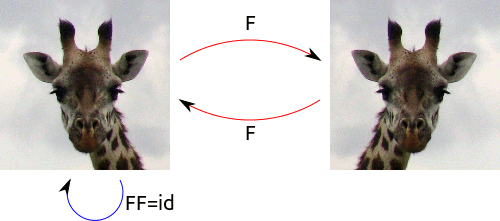$$\newcommand{\id}{\mathrm{id}}$$ $$\newcommand{\Span}{\mathrm{span}}$$ $$\newcommand{\kernel}{\mathrm{null}\,}$$ $$\newcommand{\range}{\mathrm{range}\,}$$ $$\newcommand{\RealPart}{\mathrm{Re}}$$ $$\newcommand{\ImaginaryPart}{\mathrm{Im}}$$ $$\newcommand{\Argument}{\mathrm{Arg}}$$ $$\newcommand{\norm}{\| #1 \|}$$ $$\newcommand{\inner}{\langle #1, #2 \rangle}$$ $$\newcommand{\Span}{\mathrm{span}}$$

# 2.1: Symmetry and Functions

$$\newcommand{\vecs}{\overset { \rightharpoonup} {\mathbf{#1}} }$$ $$\newcommand{\vecd}{\overset{-\!-\!\rightharpoonup}{\vphantom{a}\smash {#1}}}$$$$\newcommand{\id}{\mathrm{id}}$$ $$\newcommand{\Span}{\mathrm{span}}$$ $$\newcommand{\kernel}{\mathrm{null}\,}$$ $$\newcommand{\range}{\mathrm{range}\,}$$ $$\newcommand{\RealPart}{\mathrm{Re}}$$ $$\newcommand{\ImaginaryPart}{\mathrm{Im}}$$ $$\newcommand{\Argument}{\mathrm{Arg}}$$ $$\newcommand{\norm}{\| #1 \|}$$ $$\newcommand{\inner}{\langle #1, #2 \rangle}$$ $$\newcommand{\Span}{\mathrm{span}}$$ $$\newcommand{\id}{\mathrm{id}}$$ $$\newcommand{\Span}{\mathrm{span}}$$ $$\newcommand{\kernel}{\mathrm{null}\,}$$ $$\newcommand{\range}{\mathrm{range}\,}$$ $$\newcommand{\RealPart}{\mathrm{Re}}$$ $$\newcommand{\ImaginaryPart}{\mathrm{Im}}$$ $$\newcommand{\Argument}{\mathrm{Arg}}$$ $$\newcommand{\norm}{\| #1 \|}$$ $$\newcommand{\inner}{\langle #1, #2 \rangle}$$ $$\newcommand{\Span}{\mathrm{span}}$$

(For best results, view this video in full-screen.)

Now we will work towards a precise definition of a group. In mathematics, we often begin explorations with some general concept (in our case, symmetry) and then work on those concepts until we can arrive at a very precise definition. Having precise definitions allows us better abstractions and generalizations; the more precise our definitions, the better the mathematics we can expect to derive.

Recall our definition of the symmetries of a geometric object: all of the ways of moving that object back onto itself without changing it. Each of these different ways of moving the object back onto itself we can identify as a function. Let's call our object $$X$$. Then every symmetry of $$X$$ we can identify as a certain special function $$f:X\rightarrow X$$.

Earlier we noted that leaving $$X$$ alone should also be a symmetry of $$X$$. This is the identity function! $$id:X\rightarrow X$$, with $$id(x)=x$$ for all points $$x\in X$$.

Next, we notice that composition of functions is a helpful operation: Indeed, if we have two different symmetries $$f$$ and $$g$$ of $$X$$, then their composition $$g\circ f$$ will also be a symmetry. The first function applied to $$X$$ 'moves $$X$$ onto itself without changing it,' and then the second does as well. Thus, the composition is also a symmetry.

Finally, we note in passing that the composition of functions is associative: for three symmetries $$f,g,h$$, we have $$(f\circ g)\circ h = f\circ (g\circ h)$$.Composition of two symmetries of a rectangle.

We really need some examples here! So let's consider the perfectly symmetrical face. There are only two symmetries: the identity and the left-to-right flip (reflection over the vertical axis). Call the identity $$e$$ and the flip $$f$$. Then we see that, considered as functions from the face to itself, $$f\circ f=e$$.The red arrows describe the flip over the vertical axis. Do it twice, and you end up where you started: $$F\circ F=id$$.

Now remember our 'bumpy' hexagon, which only had rotational symmetries. Call the identity $$e$$ and let $$r$$ be the clockwise rotation by $$60^\circ$$. All of the other rotations we can think of as $$r$$ composed with itself some number of times; we'll just write this as $$r^k$$. So all of the symmetries of the bumpy hexagon are $$\{e, r, r^2, r^3, r^4, r^5\}$$. We notice that $$r^5\circ r=r^6=e$$. This is quite interesting! In fact, we can make a 'composition table' to keep track of what happens when we compose any two of the symmetries.The six rotational symmetries of the bumpy hexagon. The blue arrow represents the rotation $$r$$; $$r^6=id$$.

And now we can make a slightly less obvious observation: For any of these functions, we can find another symmetry taking the object 'back' to its original orientation. Thus, for any symmetry $$f$$, there exists a $$g$$ such that $$f\circ g=e$$. The function $$g$$ is then called the inverse of $$f$$. We've already seen two examples of this.

Write down all of the symmetries of an equilateral triangle. Make a 'composition table' of the symmetries, showing what happens when any two of them are composed. Then make a list of each symmetry and its inverse. What do you observe?

Callstack: